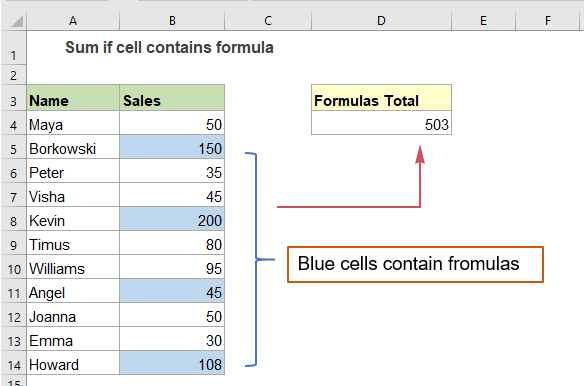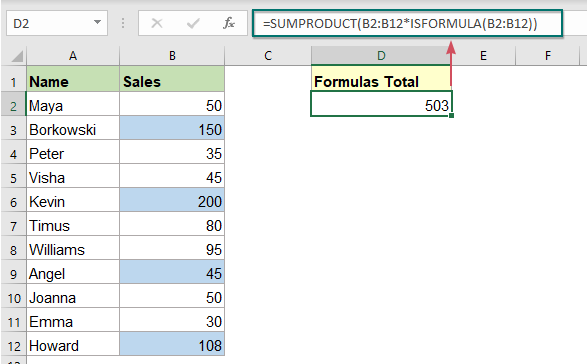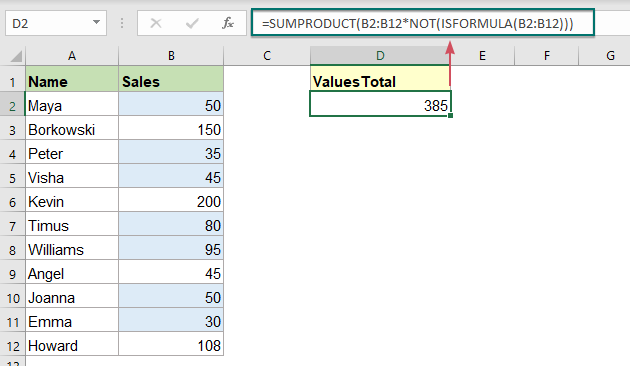## Sum only cells containing formulas in Excel

Supposing, you have a list of values in Excel, some of them are created by formulas, now, you just want to sum only the cells that contain the formulas as below screenshot shown. How could do this task in Excel?#### Sum only cells containing formulas in Excel

In Excel, you can create a simple formula based on the SUMPRODUCT and ISFORMULA functions to sum only the formula cells in a range of cells, the generic syntax is:

=SUMPRODUCT(range*ISFORMULA(range))
• range: The data range that you want to sum formula cells from.

Please enter or copy the below formula into a blank cell, and then press Enter key to get the calculation, see screenshot:

=SUMPRODUCT(B2:B12*ISFORMULA(B2:B12))##### Explanation of the formula:

=SUMPRODUCT(B2:B12*ISFORMULA(B2:B12)):

• ISFORMULA(B2:B12): This ISFORMULA function is used to test if a cell contains formula. If a cell contains a formula, it will return TRUE, and FALSE if not. So, you will get an array like this: {FALSE;TRUE;FALSE;FALSE;TRUE;FALSE;FALSE;TRUE;FALSE;FALSE;TRUE}.
• B2:B12*ISFORMULA(B2:B12)= {50;150;35;45;200;80;95;45;50;30;108}*{FALSE;TRUE;FALSE;FALSE;TRUE;FALSE;FALSE;TRUE;FALSE;FALSE;TRUE}: The math operation forces the TRUE and FALSE values to 1s and 0s. So, the multiplication of the two arrays will be like this: {50;150;35;45;200;80;95;45;50;30;108}*{0;1;0;0;1;0;0;1;0;0;1}={0;150;0;0;200;0;0;45;0;0;108}.
• SUMPRODUCT(B2:B12*ISFORMULA(B2:B12))= SUMPRODUCT({0;150;0;0;200;0;0;45;0;0;108}): At last, the SUMPRODUCT function sums all values in the array to get the result: 503.

Tips: Sum only values skip formulas

If you need to sum values while skipping the formula cells, you can add the NOT function into the above formula like this:

=SUMPRODUCT(B2:B12*NOT(ISFORMULA(B2:B12)))#### Relative function used:

• SUMPRODUCT:
• The SUMPRODUCT function can be used to multiply two or more columns or arrays together, and then get the sum of products.
• ISFORMULA:
• The Excel ISFORMULA function returns TRUE if a cell contains a formula, and FALSE if not.

#### More articles:

• Sum If Cells Contain Specific Text In Another Column
• This tutorial will show you how to sum values if cells contain specific or partial text in another column. Take the below data range as an example, to get the total amounts of the products containing the text “T-Shirt”, both the SUMIF function and SUMPRODUCT function can deal with this task in Excel.
• Sum Smallest Or Bottom N Values Based On Criteria
• In previous tutorial, we have discussed how to sum the smallest n values in a data range. In this article, we will perform a further advanced operation – to sum the lowest n values based on one or more criteria in Excel.

### The Best Office Productivity Tools

#### Kutools for Excel - Helps You To Stand Out From Crowd

 Popular Features: Find, Highlight or Identify Duplicates  |  Delete Blank Rows  |  Combine Columns or Cells without Losing Data  |  Round without Formula ... Super VLookup: Multiple Criteria  |  Multiple Value  |  Across Multi-Sheets  |  Fuzzy Lookup... Adv. Drop-down List: Easy Drop Down List  |  Dependent Drop Down List  |  Multi-select Drop Down List... Column Manager: Add a Specific Number of Columns  |  Move Columns  |  Toggle Visibility Status of Hidden Columns  |  Compare Columns to Select Same & Different Cells ... Featured Features: Grid Focus  |  Design View  |  Big Formula Bar  |  Workbook & Sheet Manager | Resource Library (Auto Text)  |  Date Picker  |  Combine Worksheets  |  Encrypt/Decrypt Cells  |  Send Emails by List  |  Super Filter  |  Special Filter (filter bold/italic/strikethrough...) ... Top 15 Toolsets:  12 Text Tools (Add Text, Remove Characters ...)  |  50+ Chart Types (Gantt Chart ...)  |  40+ Practical Formulas (Calculate age based on birthday ...)  |  19 Insertion Tools (Insert QR Code, Insert Picture from Path ...)  |  12 Conversion Tools (Numbers to Words, Currency Conversion ...)  |  7 Merge & Split Tools (Advanced Combine Rows, Split Excel Cells ...)  |  ... and more

Kutools for Excel Boasts Over 300 Features, Ensuring That What You Need is Just A Click Away...#### Office Tab - Enable Tabbed Reading and Editing in Microsoft Office (include Excel)

• One second to switch between dozens of open documents!
• Reduce hundreds of mouse clicks for you every day, say goodbye to mouse hand.
• Increases your productivity by 50% when viewing and editing multiple documents.
• Brings Efficient Tabs to Office (include Excel), Just Like Chrome, Edge and Firefox.